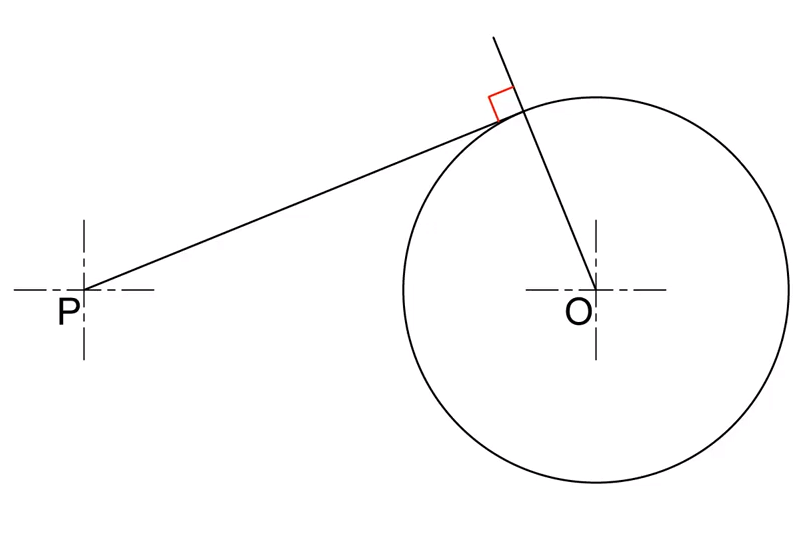## Tangent from a point to a circle

This is the simplest form of tangent to a circle. To construct this tangent you need a given point where the tangent will start from and a circle where it will touch to. The point can be anywhere around the circles, and we can have more than one point producing a tangent to a circle.The worksheets linked below provide two designs that incluide the tangents from a point to a circle construction.